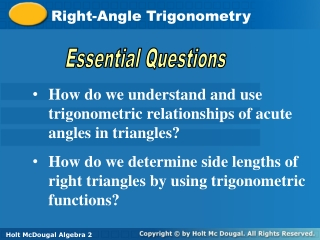Download PresentationRight-Angle Trigonometry

# Right-Angle Trigonometry

Download Presentation## Right-Angle Trigonometry

- - - - - - - - - - - - - - - - - - - - - - - - - - - E N D - - - - - - - - - - - - - - - - - - - - - - - - - - -
##### Presentation Transcript

1. Right-Angle Trigonometry Essential Questions • How do we understand and use trigonometric relationships of acute angles in triangles? • How do we determine side lengths of right triangles by using trigonometric functions? Holt McDougal Algebra 2 Holt Algebra 2

2. A trigonometric function is a function whose rule is given by a trigonometric ratio. A trigonometric ratio compares the lengths of two sides of a right triangle. The Greek letter theta θ is traditionally used to represent the measure of an acute angle in a right triangle. The values of trigonometric ratios depend upon θ.

3. SOHCAHTOA

4. Finding Trigonometric Ratios Find the value of the sine, cosine, and tangent functions for θ. hyp. opp. adj. sin θ = cos θ = tan θ =

5. Finding Trigonometric Ratios Find the value of the sine, cosine, and tangent functions for θ. hyp. adj. opp. sin θ = cos θ = tan θ =

6. You will frequently need to determine the value of trigonometric ratios for 30°,60°, and 45° angles as you solve trigonometry problems. Recall from geometry that in a 30°-60°-90° triangle, the ration of the side lengths is 1: 3 :2, and that in a 45°-45°-90° triangle, the ratio of the side lengths is 1:1: 2.

7. Substitute 30° for θ, x for opp, and 74 for hyp. ° Substitute for sin 30°. Finding Side Lengths of Special Right Triangles Use a trigonometric function to find the value of x. Which function relates the opposite and the hypotenuse? hyp. opp. 2x = 74 Solve for x. x = 37

8. ° ° Substitute 45 for θ, x for opp, and 20 for hyp. Finding Side Lengths of Special Right Triangles Use a trigonometric function to find the value of x. Which function relates the opposite and the hypotenuse? hyp. opp. Substitute for sin 45°. Solve for x.

9. Caution! Make sure that your calculator is set to interpret angle values as degrees. Press DRG. Check that Degree and not Radian is highlighted.

10. Finding Side Lengths of Other Right Triangles Use a trigonometric function to find the value of x. Which function relates the opposite and the adjacent? Substitute 38 for θ, 42 for opp., and x for adj. opp. Because x is on bottom, divide. adj.

11. Finding Side Lengths of Other Right Triangles Use a trigonometric function to find the value of x. hyp. Which function relates the adjacent and the hypotenuse? adj. Substitute 52 for θ, x for adj., and 155 for hyp. Because x is on top, multiply.

12. Lesson 10.1 Practice A